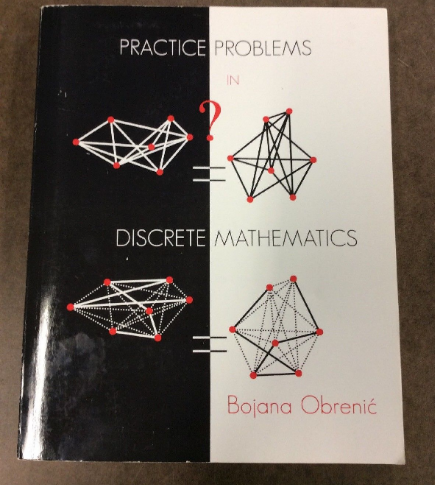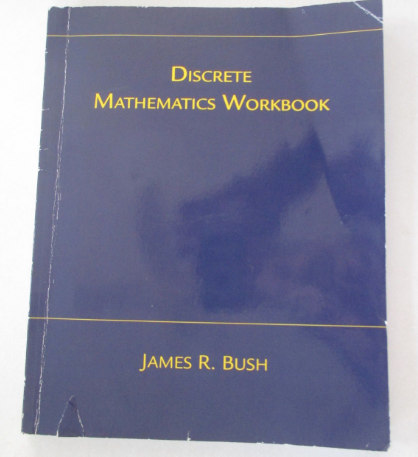# Statistics: Counting Techniques

Problem: How many words can be formed by ordering the letters ARKANSAS ?

*SPOILER*: You can watch the video above if you don’t feel like reading the full article.

First we need to count the number of letters or spots in our word ARKANSAS. The word contains 8 letters.

Now we want to look at the number ways we can arrange one of the letters in the 8 spots, let’s start with the letter ‘A’ in ARKANSAS. We first need to count the number of A’s, the number of A’s = 3. Now we can use the combination formula where n=8 and k=3.

The combination formula is
nCk = n! / ((n-k)! * k!)

So the combination formula for the A’s is nCk or 8C3, where again n=8 and k=3. More importantly if we solve the formula then 8C3 = 56, which is the number of ways we can arrange the letter A.

The number of ways we can arrange the 3 A’s in 8 spots
8C3 = 56

Now, we only have 5 spots left, because we used 3 of them for the letter ‘A’, so let’s see how we can place any two of the ‘S’ character in the remaining 5 positions. Again we must use the combination formula, but this time n= 5, and k = 2 to get 5C2.

The number of ways we can arrange the 2 S’s in 5 spots
5C2 = 10

Next let’s see how we can arrange the one ‘R’ in the remaining 3 positions. Only 3 positions remain because we have used the 2 S’s and the 3 A’s, so 5 spots out of 8 have been taken.

The number of ways we can arrange the 1 R in 3 spots
3C1 = 3

Next we want to arrange the one ‘K’ in any one of the remaining two positions.

The number of ways we can arrange the 1 K in 2 spots
2C1 = 2

Last but not least let’s place the one ’N’ in any one of the remaining one positions.

The number of ways we can arrange the 1 N in 1 spot
1C1 = 1

## CONCLUSION:

The original question asked, how many words can be formed by ordering the letters ARKANSAS ?

The answer is (56) (10) (3) (2) (1) = 3,360.

So there are 3,360 words that can be formed by ordering the letters in ARKANSAS.

Below, you can watch another video on counting techniques !

If you want to read up on more combinatoric problems like the one in this article or Discrete Math topics in general a great book to easily learn and practice these topics is Practice Problems in Discrete Mathematics by Bojana Obrenic’, and Discrete Math Workbook: Interactive Exercises by James R. bush.Practice Problems in Discrete Mathematics by Bojana Obrenic’Discrete Math Workbook: Interactive Exercises by James R. bush

Thanks for reading this article I hope its helpful to you all ! If you enjoyed this article and found it helpful please leave some claps to show your appretiation. Keep up the learning, and if you would like more mathematics, computer science, programming and algorithm analysis videos please visit and subscribe to my YouTube channels (randerson112358 & compsci112358 )

# Check Out the following for content / videos on Computer Science, Algorithm Analysis, Programming and Logic:

compsci112358:

Video Tutorials on Recurrence Relation:

Video Tutorial on Algorithm Analysis:
https://www.udemy.com/algorithm-analysis/